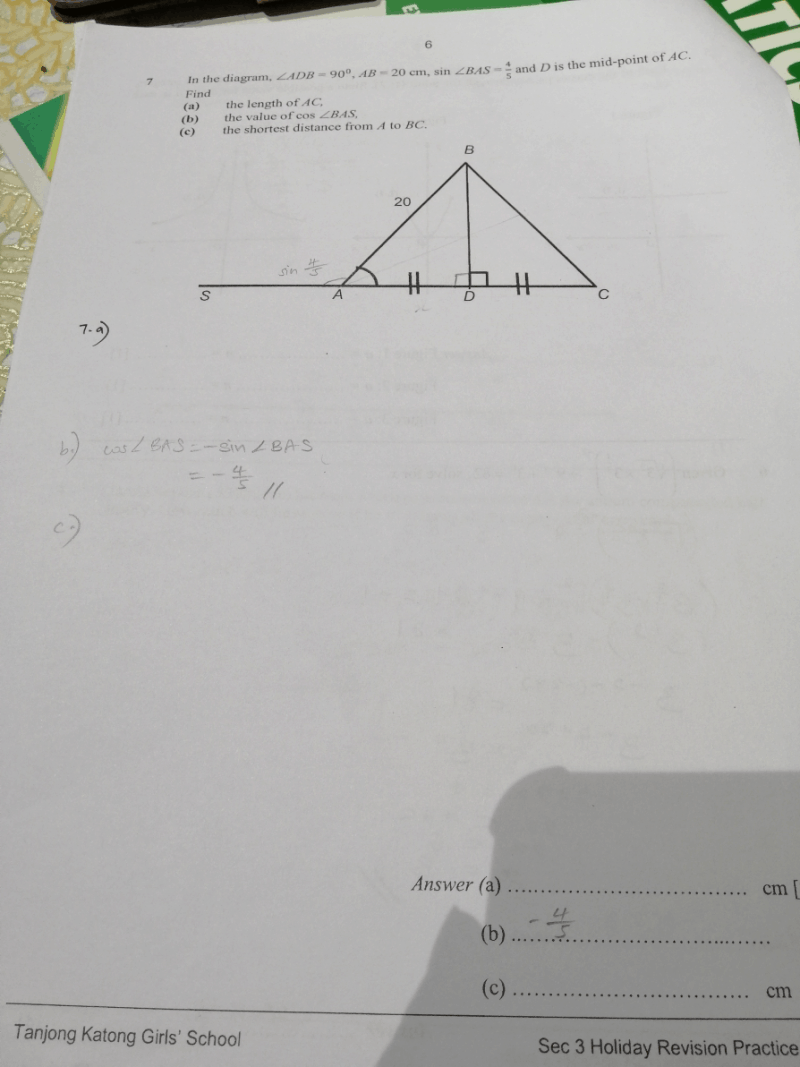# Questiona) sin θ° = sin (180 – θ)°
∴ sin ∠BAS = sin ∠BAD = 4/5
BD/AB = 4/5
BD/20 = 4/5
BD = 16

= √144
= 12

Since D is mid-point of AC, AC = 12 x 2 = 24 cm

b) cos (180° – θ) = -cos θ
∴ cos ∠BAS = – cos ∠BAD = -16/20 = -5/4

c) Using Law of Cosines,
242 = 202 + 202 – 2 x 20 x 20 x cos ∠ABC
576 = 400 + 400 – 800 cos ∠ABC
576 = 800 – 800 cos
∠ABC
800 cos ∠ABC = -224
cos
∠ABC = -224/800 = -7/25
∠ABC = cos-1 (-7/25)
≈ 106.26°

Let h = shortest distance from A to BC (height of triangle ABC with BC as base)

sin ABC = h/AB
sin 106.26° = h/20
h = 20 x sin 106.26°
19.20 cm

0 Replies 0 Likes